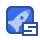# CSE121-Project-Matrix-Calculator：矩阵计算器-源码

VIP专享 2021-02-17 02:54:48 4KB ZIP14收藏

CSE-121-项目 一个c ++程序，最多接受100x100个矩阵以对其执行多个操作。 这些操作包括： 1. Addition and Subtraction 2. Multiplication and division 3. Finding the Transpose matrix 4. Raising a matrix to an exponent 5. Finding the determinant for a matrix 6. Finding the inverse of the matrix 请注意，计算器对以下格式的矩阵进行运算： [ a b c; d e f; g h i;] where this matrix is a 3 by 3 square matrix. The system check if the input matrix was given corre

...展开详情

• 4614
资源
• 7
粉丝
•等级
•至尊王者CSE121-Project-Matrix-Calculator：矩阵计算器-源码 (VIP专享) VIP下载
1/0